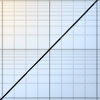#### You may also like### Area L

By sketching a graph of a continuous increasing function, can you prove a useful result about integrals?### Integral Equation

Solve this integral equation.### Integral Sandwich

Generalise this inequality involving integrals.

# Integral Arranging

##### Age 16 to 18Challenge Level

How might you sort these integrals into an order or different groups?

$$\int\frac{1}{1+x^2}\rm dx\quad\quad\int\frac{1}{1-x^2}\,dx$$ $$\int\frac{1}{(1+x)^2}\,dx\quad\quad\int\frac{1}{(1-x)^2}\,dx$$ $$\int\frac{1}{1+x}\,dx\quad\quad\int\frac{1}{1-x}\,dx$$ $$\int\frac{1}{\sqrt{1+x^2}}\,dx\quad\quad\int\frac{1}{\sqrt{1-x}}\,dx$$ $$\int{\sqrt{1+x^2}}\,dx\quad\quad\int{\sqrt{1-x^2}}\,dx$$ $$\int \sqrt{1+x}dx\quad\quad\int \sqrt{1-x}\,dx$$

Did you know ... ?

Although you can compute many integrals using Wolfram's integrator, if you do enough mathematics you will realise that the class of functions which integrate to a closed algebraic form is, by most ways of counting, small. There are many advanced analytical tools which allow for the manipulation and approximate computation of integrals more generally. A large part of this procedure involves classifying integrals into different types before suitable approximations are made.